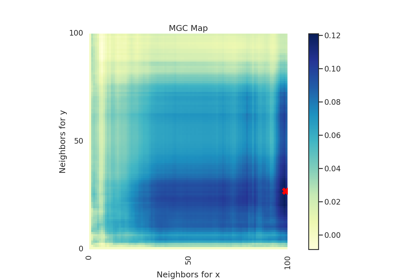RV¶

class hyppo.independence.RV

Rank Value (RV) test statistic and p-value.

RV is the multivariate generalization of the squared Pearson correlation coefficient 1. The RV coefficient can be thought to be closely related to principal component analysis (PCA), canonical correlation analysis (CCA), multivariate regression, and statistical classification 1.

Notes

The statistic can be derived as follows 1 2:

Let $$x$$ and $$y$$ be $$(n, p)$$ samples of random variables $$X$$ and $$Y$$. We can center $$x$$ and $$y$$ and then calculate the sample covariance matrix $$\hat{\Sigma}_{xy} = x^T y$$ and the variance matrices for $$x$$ and $$y$$ are defined similarly. Then, the RV test statistic is found by calculating

$\mathrm{RV}_n (x, y) = \frac{\mathrm{tr} \left( \hat{\Sigma}_{xy} \hat{\Sigma}_{yx} \right)} {\mathrm{tr} \left( \hat{\Sigma}_{xx}^2 \right) \mathrm{tr} \left( \hat{\Sigma}_{yy}^2 \right)}$

where $$\mathrm{tr} (\cdot)$$ is the trace operator.

The p-value returned is calculated using a permutation test using hyppo.tools.perm_test.

References

1(1,2,3)

P. Robert and Y. Escoufier. A Unifying Tool for Linear Multivariate Statistical Methods: The RV- Coefficient. Journal of the Royal Statistical Society. Series C (Applied Statistics), 25(3):257–265, 1976. doi:10.2307/2347233.

2

Yves Escoufier. Le Traitement des Variables Vectorielles. Biometrics, 29(4):751–760, 1973. doi:10.2307/2529140.

Methods Summary

 RV.statistic(x, y) Helper function that calculates the RV test statistic. RV.test(x, y[, reps, workers, random_state]) Calculates the RV test statistic and p-value.

RV.statistic(x, y)

Helper function that calculates the RV test statistic.

Parameters

x,y (ndarray) -- Input data matrices. x and y must have the same number of samples and dimensions. That is, the shapes must be (n, p) where n is the number of samples and p is the number of dimensions.

Returns

stat (float) -- The computed RV statistic.

RV.test(x, y, reps=1000, workers=1, random_state=None)

Calculates the RV test statistic and p-value.

Parameters
• x,y (ndarray) -- Input data matrices. x and y must have the same number of samples and dimensions. That is, the shapes must be (n, p) where n is the number of samples and p is the number of dimensions.

• reps (int, default: 1000) -- The number of replications used to estimate the null distribution when using the permutation test used to calculate the p-value.

• workers (int, default: 1) -- The number of cores to parallelize the p-value computation over. Supply -1 to use all cores available to the Process.

Returns

• stat (float) -- The computed RV statistic.

• pvalue (float) -- The computed RV p-value.

Examples

>>> import numpy as np
>>> from hyppo.independence import RV
>>> x = np.arange(7)
>>> y = x
>>> stat, pvalue = RV().test(x, y)
>>> '%.1f, %.2f' % (stat, pvalue)
'1.0, 0.00'

Examples using hyppo.independence.RV¶